Published: 21 October 2017

# A new type of high frequency mechanical vibration table

Jin Gao1
Chaowei Li2
Dongying Wang3
Wenbin Du4
Shili Li5
Qiang Lei6
1, 2, 3, 4, 5, 6Northwest Institute of Mechanical and Electrical Engineering, 712099, Xianyang, P. R. China
Corresponding Author:
Jin Gao
Views 597

#### Abstract

This paper puts forward a new type of mechanical vibration table. First of all, the structure and working principle of this vibration table is described. Then the kinetic equation and the three-dimensional model of the eccentric exciter are established, the simulation experiments are carried out, and the displacement curves, velocity curves and acceleration time curves at different frequencies are analyzed. Finally, finite element analysis of eccentric wheels is carried out based on solidwork2012. The simulation results show that the mechanical vibration of this new mechanical vibration table is achievable at 0-100 Hz.

## 1. Introduction

Vibration testing is not only a basic test in modern industry, but also an important means of product development. It is widely used in many important engineering areas, such as environmental testing of satellites and rockets, road simulation tests for cars and walking machinery, and so on [1-3]. As the standard equipment of vibration experiment, the performance of vibration table directly affects the technological progress and development of various fields. At the same time it is also an important indicator of national industrial development [4, 5]. Due to the limitation of mechanical structure, the traditional mechanical vibration table has the following problems: the work frequency range is small, the acceleration waveform distortion is big, and the noise is big and so on.

The purpose of this paper is to propose a new type of eccentric wheeled mechanical vibration table. Based on SolidWorks2012, the mathematical model was established and the kinematic simulation experiment was carried out. And feasibility of the scheme is verified by finite element analysis of the eccentric wheel.

## 2. Basic structure and working principle of the eccentric wheeled vibration table

Fig. 1 shows the eccentric wheel mechanical vibration table. It includes four parts: the fixed supporting part of the vibration table, vibration excitation part, vibration body part, and peripheral component section.

Fig. 1Vibration table system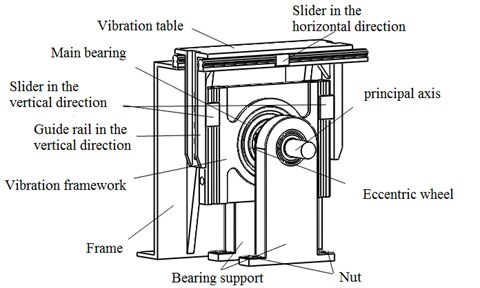As shown in the Fig. 1, the eccentric wheel is the vibration source for the entire system. The balance wheel is intended to ensure the dynamic balance of the eccentric wheel at high speed. Since the eccentric wheel has an eccentricity $e$, when the spindle is driven by the motor, the eccentric wheel rotates around the center of the spindle. And he vibrating frame is moved in both horizontal and vertical directions. Driven by the eccentric wheel, the vibrating frame is moved in both horizontal and vertical directions. Since the vibration table is connected to the vibration frame in the vertical direction by the slider, the vibration table only output horizontal vibration.

Assuming that when the eccentric wheel rotation center and the geometric center in the same horizontal position as the initial state. At this point, the vibration table is at the maximum amplitude of $+e$. Then, the eccentric wheel is driven by the spindle to the maximum amplitude-$e$ in the opposite direction. This process is a working cycle of the vibration table. From the above analysis we can see that vibration frequency of the table is determined by the principal axis speed, and the maximum amplitude is determined by the eccentric eccentricity.

## 3. Calculation of vibrating body

The movement relationship of eccentric wheel is shown in Fig. 2.

Fig. 2Eccentric movement relationship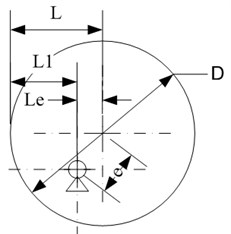From Fig. 2 we can draw:

1
${L}_{1}=\frac{d}{2}-e\mathrm{c}\mathrm{o}\mathrm{s}\omega t.$

Find the derivative of time for Eq. (1), then in the horizontal direction:

Speed is:

2
$v={{L}_{1}}^{\text{'}}=e\omega \mathrm{s}\mathrm{i}\mathrm{n}\omega t.$

Acceleration is:

3
$a=e{\omega }^{2}\mathrm{c}\mathrm{o}\mathrm{s}\omega t.$

From the Eq. (2), Eq. (3) we can see that the speed, acceleration are sinusoidal changed. It is suitable for use as a source of vibration

### 3.1. System vibration model

The external force of the vibration table system is the simple harmonic excitation force, that is $F=A\mathrm{s}\mathrm{i}\mathrm{n}\omega t$. According to the D’Alembert’s principle, the vibration equation of the system can be described as:

4
$Mx\text{'}\text{'}+{C}_{x}x\text{'}+{K}_{x}x={m}_{0}r\left(\omega t{\right)}^{2}\mathrm{s}\mathrm{i}\mathrm{n}\omega t,$
5
$My\text{'}\text{'}+{C}_{y}y\text{'}+{K}_{y}y={m}_{0}r\left(\omega t{\right)}^{2}\mathrm{c}\mathrm{o}\mathrm{s}\left(\omega t\right),$

where $M$ – the total mass of vibrator; $x$, $y$ – the displacement of the vibrator in the $x$, $y$ direction; $x\text{'}$, $y\text{'}$ – the velocity of the vibrator in the $x$, $y$ direction; ${m}_{0}$ – eccentric mass; $x\text{'}\text{'}$, $y\text{'}\text{'}$ – the acceleration of the vibrator in the $x$, $y$ direction; $r$ – eccentricity; ${C}_{x}$, ${C}_{y}$ – the damping coefficient of the vibrator in the $x$, $y$ direction; ${K}_{x}$, ${K}_{y}$ – the stiffness coefficient of the vibrator in the $x$, $y$ direction.

Since $y=$ 0, then the vibration equation of the system is:

6
$Mx\text{'}\text{'}+{C}_{x}x\text{'}+{K}_{x}x={m}_{0}r\left(\omega t{\right)}^{2}\mathrm{s}\mathrm{i}\mathrm{n}\omega t.$

## 4. Virtual prototype modeling and motion simulation analysis based on SolidWorks environment

### 4.1. The establishment of virtual prototype model

In this paper, the virtual prototyping technology is used to verify the mathematical and dynamic models of the vibration table. SolidWorks is used to build the three-dimensional model.

The name of the parts and related physical properties are set, the effective constraints of contact and drive, etc. are added, and virtual prototype model of the vibration table is established which is shown in Fig. 3. The model will be used for performance simulation analysis of displacement, acceleration.

Fig. 3Vibration table virtual prototype model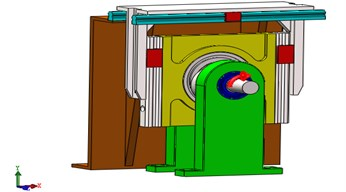### 4.2. Vibration waveform analysis

After checking its physical properties, effective constraints and loads, the vibration waveforms at a certain frequency are obtained by simulation.

As the operating frequency of vibration table is gradually increased to its maximum. So, we choose the vibration table simulation frequency of 20 Hz, 60 Hz, 100 Hz, and compare these waveforms.

Fig. 4Vibration waveform in 20 Hz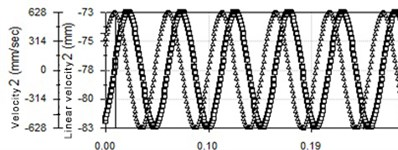a) Displacement and velocity time curve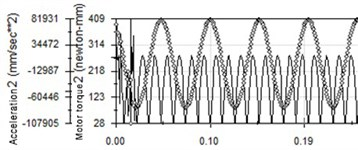b) Acceleration and principal axis

By analyzing the displacement, velocity, acceleration and torque curves of the three sets of simulation experiments, it is possible to obtain that displacement and speed waveform is good when the vibration table at a lower speed. And there will be no dislocation and jump phenomenon. With the increasing of the speed, the acceleration and torque time images have some changes.

(a) In the beginning of the vibration, there is a certain fluctuation of acceleration waveform. This is because the motor power output gradually increased, then the amplitude and frequency of the acceleration gradually increased.

(b) After a brief start-up phase, the vibration table enters a smooth working phase. The vibration curves are good.

(c) Due to the existence of non-linear behavior and parts installation gap, making the higher harmonics and the random wave are superimposed on the fundamental wave of the vibration acceleration.

Fig. 5Vibration waveform in 60 Hz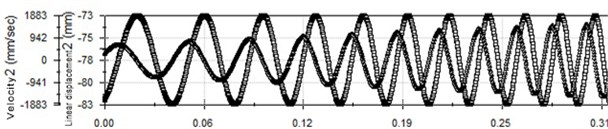a) Displacement and velocity time curve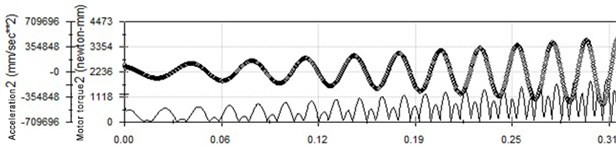b) Acceleration and principal axis

Fig. 6Vibration waveform in 100 Hz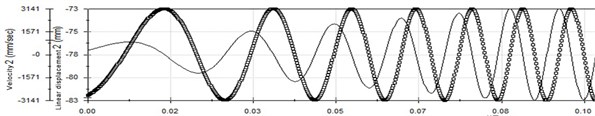a) Displacement and velocity time curve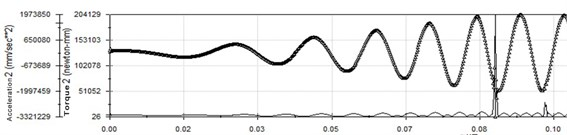b) Acceleration and principal axis

## 5. The finite element analysis of the eccentric load bearing components

In this paper, the finite element analysis of eccentric wheels and workstations is carried out. For SolidWorks Simulation, the following procedure is generally followed: Example creation → Material use → Constraint addition → Load application → Grid division → Operation analysis → Result analysis. Create an example with the mathematical model of the eccentric wheel and the table body, add the bearing constraint, and apply the torque grid as shown in Fig. 7.

The stress distribution and strain figure of the model are obtained by analyzing the arithmetic examples, as shown in Fig. 8 and Fig. 9.

It can be clearly seen from the above diagram that eccentric stress is mainly distributed in eccentric wheel and shaft connected area. The maximum stress of the eccentric wheel is 11,384,998N/m2, equivalent to 11.385 MPa, and the yield limit of the material is 241.275 MPa. So, the maximum stress of the eccentric wheel is much smaller than the yield limit of the material. Fig. 10 shows the eccentric wheel strain distribution, corresponding to the stress distribution area.

Fig. 7Meshing of eccentric wheels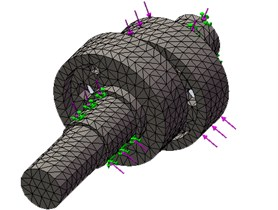Fig. 8Eccentricity stress distribution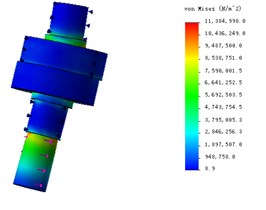Fig. 9Eccentric wheel strain figure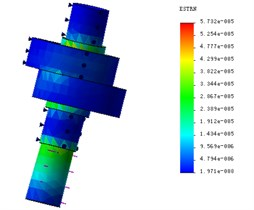## 6. Conclusions

In this paper, a new type of mechanical vibration table is proposed, which is outputted by the rotary motion of the eccentric wheel. In this paper, working principle is given, the dynamic equation of the vibrator and the system vibration model is established. Then the three-dimensional model is established and the simulation test is carried on. We obtained the waveform of the vibration table at different frequencies, and verified that the new eccentric mechanical vibration table had a good vibration waveform when the frequency was less than 100 Hz. It is proved that the scheme of the vibration table is feasible.